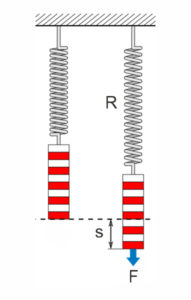The spring force – also known as the tension force – arises when an elastic body is pulled apart or compressed – for example one Metal spring made of spring steel wire . An opposing force acts in it, which returns the spring to its original position.

## Hookese’s Law

The elastic force of the body changes with expansion or compression. At Standard designs (cylindrical) consists of a linear relationship between expansion and force . This linear-elastic behavior of solids is also called Hooke’s law designated. It was named after the English scholar Robert Hooke. In general, Hooke’s law represents the linear special case in the law of elasticity.

It can be stated that the longer the distance “s” by which a spring is stretched or compressed, the stronger the counteracting spring force “F” of the spring.

## Formula for calculating the spring force:

The spring force can be calculated using the following formula:• F = Spring Force [N]
• R = spring rate [N/mm]
• s = spring deflection [mm]

Why is the spring rate negative? The minus sign in the equation means that, relative to the resting position, the direction of deflection of a spring is opposite to the spring force.

Formula for spring force without specifying the spring rate (R)The spring force formula is used not only for Compression springs,Extension springs and Torsion springs, but also for other elastic bodies. An important topic is therefore spring force in mechanics and materials technology, among other things.

## The spring rate

The spring constant or spring rate “R” depends on the material and the design of the spring. With increasing thickness or a tighter winding of the wire used, the spring constant of a helical spring increases. It is specified in the unit Newton per millimeter (N / mm) and is the quotient of the spring force “F” and the spring deflection “s”. The spring constant can also be calculated by simply changing the formula for calculating the spring force:

##• F = Spring Force [N]
• R = Spring rate / Spring constant [N/mm]
• s = spring deflection [mm]

Formula for spring constant without specifying the spring force (F) and spring deflection (s)All formulas for review and for proof of function the spring force, the spring constant and the spring deflection for torsion springs for spring torque, spring torque rate are available here for Compression springs , Extension springs and Torsion springs .

For the interpretation of a suitable Compression- , Extension- or Torsion spring please contact our technical department directly by phone (+49) 035 877 227-13 or service@gutekunst-co.com .